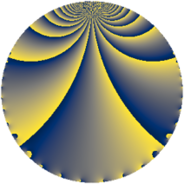# Properties

 Label 363.3.kLevel $363$ Weight $3$ Character orbit 363.k Rep. character $\chi_{363}(10,\cdot)$ Character field $\Q(\zeta_{22})$ Dimension $440$ Newform subspaces $1$ Sturm bound $132$ Trace bound $0$

# Related objects

## Defining parameters

 Level: $$N$$ $$=$$ $$363 = 3 \cdot 11^{2}$$ Weight: $$k$$ $$=$$ $$3$$ Character orbit: $$[\chi]$$ $$=$$ 363.k (of order $$22$$ and degree $$10$$) Character conductor: $$\operatorname{cond}(\chi)$$ $$=$$ $$121$$ Character field: $$\Q(\zeta_{22})$$ Newform subspaces: $$1$$ Sturm bound: $$132$$ Trace bound: $$0$$

## Dimensions

The following table gives the dimensions of various subspaces of $$M_{3}(363, [\chi])$$.

Total New Old
Modular forms 900 440 460
Cusp forms 860 440 420
Eisenstein series 40 0 40

## Trace form

 $$440 q + 100 q^{4} - 4 q^{5} + 1320 q^{9} + O(q^{10})$$ $$440 q + 100 q^{4} - 4 q^{5} + 1320 q^{9} - 88 q^{10} + 90 q^{11} - 24 q^{12} - 88 q^{13} + 68 q^{14} + 36 q^{15} - 188 q^{16} + 52 q^{20} - 76 q^{22} - 252 q^{23} - 212 q^{25} - 164 q^{26} - 78 q^{31} + 12 q^{33} - 112 q^{34} + 300 q^{36} - 150 q^{37} + 152 q^{38} - 60 q^{42} - 162 q^{44} - 12 q^{45} - 64 q^{47} + 144 q^{48} + 244 q^{49} - 2288 q^{50} + 286 q^{52} - 312 q^{53} + 296 q^{55} + 1058 q^{56} + 594 q^{58} - 76 q^{59} - 240 q^{60} - 1210 q^{62} + 240 q^{64} + 462 q^{65} - 192 q^{66} + 50 q^{67} + 12 q^{69} - 444 q^{70} - 524 q^{71} - 286 q^{73} + 120 q^{75} - 3234 q^{76} + 180 q^{77} + 450 q^{78} + 572 q^{79} - 296 q^{80} + 3960 q^{81} - 708 q^{82} - 792 q^{85} + 188 q^{86} - 1188 q^{88} - 932 q^{89} - 264 q^{90} - 738 q^{91} + 1512 q^{92} - 240 q^{93} + 792 q^{96} + 938 q^{97} - 1232 q^{98} + 270 q^{99} + O(q^{100})$$

## Decomposition of $$S_{3}^{\mathrm{new}}(363, [\chi])$$ into newform subspaces

Label Dim $A$ Field CM Traces $q$-expansion
$a_{2}$ $a_{3}$ $a_{5}$ $a_{7}$
363.3.k.a $440$ $9.891$ None $$0$$ $$0$$ $$-4$$ $$0$$

## Decomposition of $$S_{3}^{\mathrm{old}}(363, [\chi])$$ into lower level spaces

$$S_{3}^{\mathrm{old}}(363, [\chi]) \cong$$ $$S_{3}^{\mathrm{new}}(121, [\chi])$$$$^{\oplus 2}$$